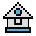Home

# ERROR Statement

#### Purpose:

To simulate the occurrence of an error, or to allow the user to define error codes.

#### Syntax:

`ERROR integer expression`

The value of integer expression must be greater than 0 and less than 255.

If the value of integer expression equals an error code already in use by GW-BASIC, the ERROR statement simulates the occurrence of that error, and the corresponding error message is printed.

A user-defined error code must use a value greater than any used by the GW- BASIC error codes. There are 76 GW-BASIC error codes at present. It is preferable to use a code number high enough to remain valid when more error codes are added to GW-BASIC.

User-defined error codes may be used in an error-trapping routine.

If an ERROR statement specifies a code for which no error message has been defined, GW-BASIC responds with the message "Unprintable Error".

Execution of an ERROR statement for which there is no error-trapping routine causes an error message to be printed and execution to halt.

For a complete list of the error codes and messages already defined in GW-BASIC, refer to Appendix A in the GW-BASIC User's Guide.

#### Examples:

The following examples simulate error 15 (the code for "String too long"):

```10 S=10
20 T=5
30 ERROR S+T
40 END
RUN
ĀString too long in 30```

Or, in direct mode:

```ERROR 15          (you type this line)
ĀString too long  (GW-BASIC types this line)```

The following example includes a user-defined error code message.

```.
.
.
110 ON ERROR GOTO 400
120 INPUT "WHAT IS YOUR BET";B
130 IF B>5000 THEN ERROR 210
.
.
.
400 IF ERR=210 THEN PRINT "HOUSE LIMIT IS \$5000"
410 IF ERL=130 THEN RESUME 120
.
.
.```